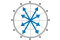# compass

Arrows emanating from origin

•## Syntax

``compass(U,V)``
``compass(Z)``
``compass(___,LineSpec)``
``compass(ax,___)``
``c = compass(___)``

## Description

example

````compass(U,V)` plots arrows originating from the point (0, 0). Specify the direction of arrows using the Cartesian coordinates `U` and `V`, with `U` indicating the x-coordinates and `V` indicating the y-coordinates. The number of arrows matches the number of elements in `U`.The `compass` function plots arrows on a circular grid with theta-axis and r-axis tick labels within an `Axes` object. Therefore, the coordinates you specify do not match the labels displayed on the plot. ```

example

````compass(Z)` plots arrows using the real and imaginary parts of the complex values specified by `Z`, with the real part indicating the x-coordinates and the imaginary part indicating the y-coordinates. This syntax is equivalent to `compass(real(Z),imag(Z))`.```

example

````compass(___,LineSpec)` sets the line style, marker symbol, and color for the arrows.```

example

````compass(ax,___)` plots arrows in the specified axes instead of the current axes.```

example

````c = compass(___)` returns a vector of `Line` objects. This syntax is useful for controlling the appearance of arrows.```

## Examples

collapse all

Create a compass plot by specifying the Cartesian coordinates of each arrow.

```u = [5 3 -4 -3 5]; v = [1 5 3 -2 -6]; compass(u,v)```Create a compass plot using polar coordinates by first converting them to Cartesian coordinates.

To do this, specify data using polar coordinates. Convert them to Cartesian coordinates using the `pol2cart` function. Then, create the plot.

```th = linspace(pi/4,2*pi,10); r = linspace(5,20,10); [u,v] = pol2cart(th,r); compass(u,v)```Note that the theta-axis and r-axis tick labels correspond to the polar coordinates.

Sample a sinusoid at equally spaced intervals. Then, compute the 10-point discrete Fourier transform of the sinusoid. The result is a vector of complex values.

```t = linspace(0,8*pi,100); y = sin(2*t) + 2*sin(t+pi/2); f = fft(y,10);```

Display the complex values using a compass plot. The real part determines the x-coordinate of each arrow, and the imaginary part determines the y-coordinate.

`compass(f)`Create a compass plot with red arrows.

```u = [5 3 -4 -3 5]; v = [1 5 3 -2 -6]; compass(u,v,'r')```Specify the line width and color of a single arrow by assigning the arrow to a variable and then setting its properties. To do this, first create a compass plot and return an array of `Line` objects.

```u = [3 5 -4 -3 5]; v = [5 1 3 -2 -6]; c = compass(u,v);```

Assign the first arrow to a variable. The first arrow corresponds to the first elements of `u` and `v`. Then, change the line width and color.

```c1 = c(1); c1.LineWidth = 2; c1.Color = 'r';```Rotate a compass plot so that 0 degrees points up by using the `view` function.

To do this, create a compass plot using polar coordinates. Convert the polar coordinates to Cartesian coordinates by using the `pol2cart` function, and then plot the converted coordinates.

```th = linspace(0,3*pi/2,10); r = linspace(5,20,10); [u,v] = pol2cart(th,r); compass(u,v)```Note that 0 degrees points to the right. Rotate the theta-axis 90 degrees in a counterclockwise direction by calling `view` and specifying the first argument as -`90`. Maintain the 2-D view by specifying the second argument as `90`.

`view(-90,90)`Note that 0 degrees now points up.

Starting in R2019b, you can display a tiling of plots using the `tiledlayout` and `nexttile` functions. Call the `tiledlayout` function to create a 1-by-2 tiled chart layout. Call the `nexttile` function to create an axes object and return the object as `ax1`. Create the left plot by passing `ax1` to the `compass` function. Add a title to the plot by passing the axes to the `title` function. Repeat the process to create the right plot.

```u = [7 5 -2 -5 8]; tiledlayout(1,2) % Left plot ax1 = nexttile; v1 = [3 7 5 -4 -6]; compass(ax1,u,v1) title(ax1,'Left Plot') % Right plot ax2 = nexttile; v2 = [-3 -4 -5 6 6]; compass(ax2,u,v2) title(ax2,'Right Plot')```## Input Arguments

collapse all

x-coordinates, specified as a scalar, vector, or matrix. Specify Cartesian values. To convert data from polar to Cartesian, use `pol2cart`.

The size of `U` must match the size of `V`.

y-coordinates, specified as a scalar, vector, or matrix. Specify Cartesian values. To convert data from polar to Cartesian, use `pol2cart`.

The size of `V` must match the size of `U`.

Complex values, specified as a scalar, vector, or matrix. The real part of `Z` indicates the x-coordinates of arrows, and the imaginary part indicates the y-coordinates.

Line style, marker, and color, specified as a character vector or string containing symbols. The symbols can appear in any order. You do not need to specify all three characteristics (line style, marker, and color). For example, if you omit the line style and specify the marker, then the plot shows only the marker and no line.

Example: `'--or'` is a red dashed line with circle markers

Line StyleDescriptionResulting Line
`'-'`Solid line`'--'`Dashed line`':'`Dotted line`'-.'`Dash-dotted lineMarkerDescriptionResulting Marker
`'o'`Circle`'+'`Plus sign`'*'`Asterisk`'.'`Point`'x'`Cross`'_'`Horizontal line`'|'`Vertical line`'s'`Square`'d'`Diamond`'^'`Upward-pointing triangle`'v'`Downward-pointing triangle`'>'`Right-pointing triangle`'<'`Left-pointing triangle`'p'`Pentagram`'h'`HexagramColor NameShort NameRGB TripletAppearance
`'red'``'r'``[1 0 0]``'green'``'g'``[0 1 0]``'blue'``'b'``[0 0 1]``'cyan'` `'c'``[0 1 1]``'magenta'``'m'``[1 0 1]``'yellow'``'y'``[1 1 0]``'black'``'k'``[0 0 0]``'white'``'w'``[1 1 1]`Target axes, specified as an `Axes` object.

## Version History

Introduced before R2006a